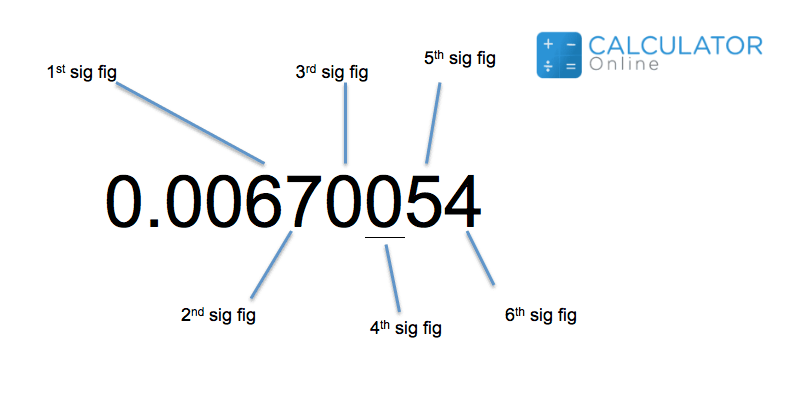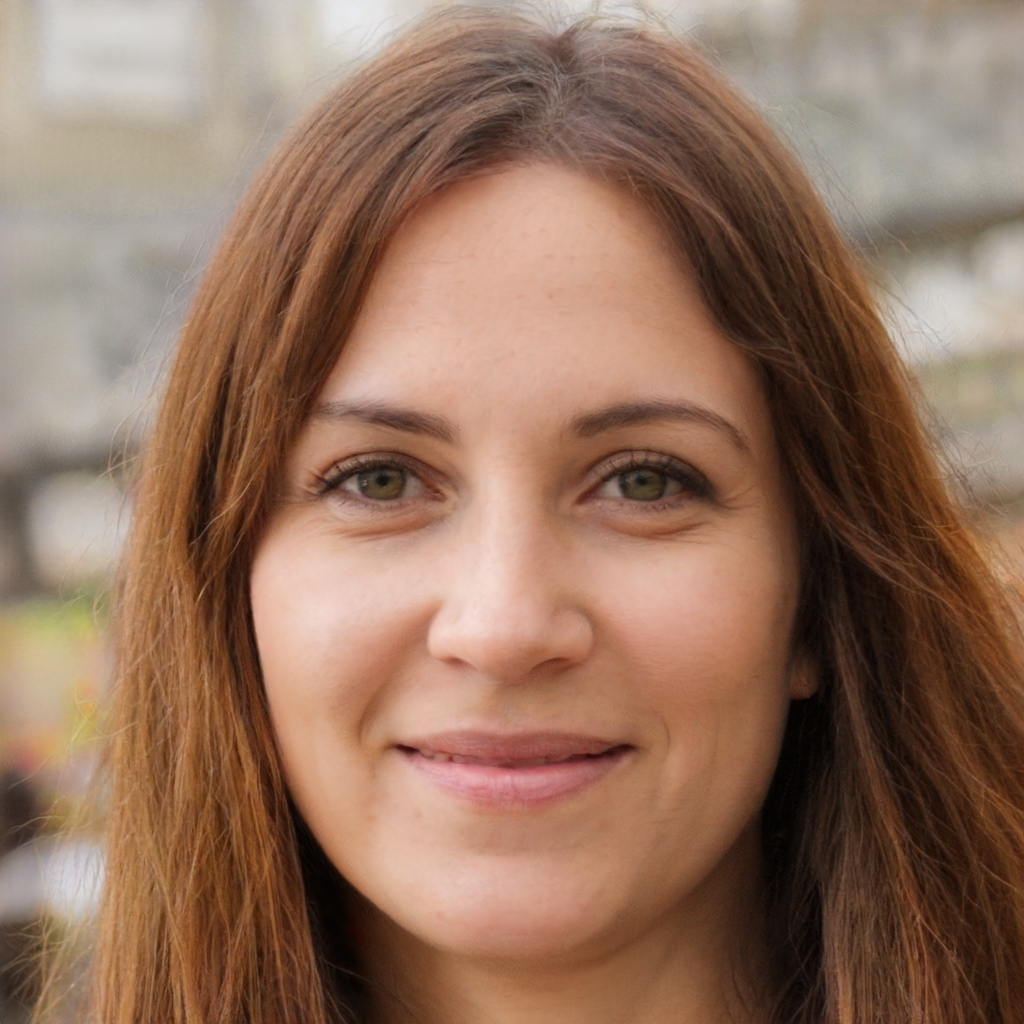Uh Oh! It seems you’re using an Ad blocker!

We always struggled to serve you with the best online calculations, thus, there's a humble request to either disable the AD blocker or go with premium plans to use the AD-Free version for calculators.

Or

Blog / Education / Understand Rounding And Significant Figures# Understand Rounding And Significant Figures

Well, rounding is the term that takes into account to round an appropriate number of significant figures. Sig figs are the digits that indicate useful information about the accuracy of a measurement. Also, they are sometimes referred to as ‘significant digits.’ So, give a read to this blog post to know more about the rounding sig figs and how many sig figs a number has and even significant figures examples with answers. Additionally, use a sig figs calculator to get numbers in sig fig.

## How to Count Significant Digits?

Here are the some examples of how many significant figures an expression has:

3.14167 have six significant digits as it is said to that all the digits give useful information.

What about 1000, it has only one significant figure, only the 1 is interesting that indicate anything specific. Here, we don’t sure about the hundreds, tens, or units place. The zeros may just said to be placeholders, and even may have rounded something off to get this value.

So, what about 1000.0, the expression have five significant figures. The “.0” is interesting that shows the presume accuracy of the measurement being made. It tells that the measurement is accurate to the tenths place, but that there happen to be zero tenths.

0.00035 consists of two significant digits. Only the 3 and 5 indicates something, the remaining zeroes are said to be placeholders, and just providing information about relative size. Also, you can add this expression into the significant figures calculator to know how many sig figs this expression contains.

Well, here is another rounding examples, remember that each number is rounded to four, three, and two significant digits:

• Let, Round 742,396 to four, three, and two sig figs:

To do rounding, you just have to begin with the first significant digit, which is the 7. Then, you ought to count to the right form there. The first four sig figs of 742,396 are the 7, the 4, the 2, and the 3. And, just to the right of the 3 is a 9; this value is “5 or greater”, so, you have to round the 3 up to 4. You have to replace the remaining digits (the 9 and the 6) with zeroes. Then:

742,400 (four significant digits)

To round 742,396 to three places, you ought to begin again with the 7 and include the next two digits, that being the 4 and the 2. Since the very next digit is a 3, which is “less than 5”, you ought to leave the 2 alone, and don’t round up. You have to replace the three digits after the comma with zeroes. Then:

742,000 (three significant digits)

To round 742,396 to two places, you ought to use only the first two digits that are followed by a 2, so no need to round up. Instead, you just have to replace the final four digits with zeroes, to get: 740,000 (two significant digits)### Jacquelin Smith

Last updated: November 28, 2022

Jacquelin Smith is an accomplished writer and a Researcher; She has done MS(Computer Science) and doing content writing for since 2019. She is an ardent writer and expert in Niches like Digital Marketing, Social media, Mathematics, etc. She is an expert writer and commentator at the same time.

Search